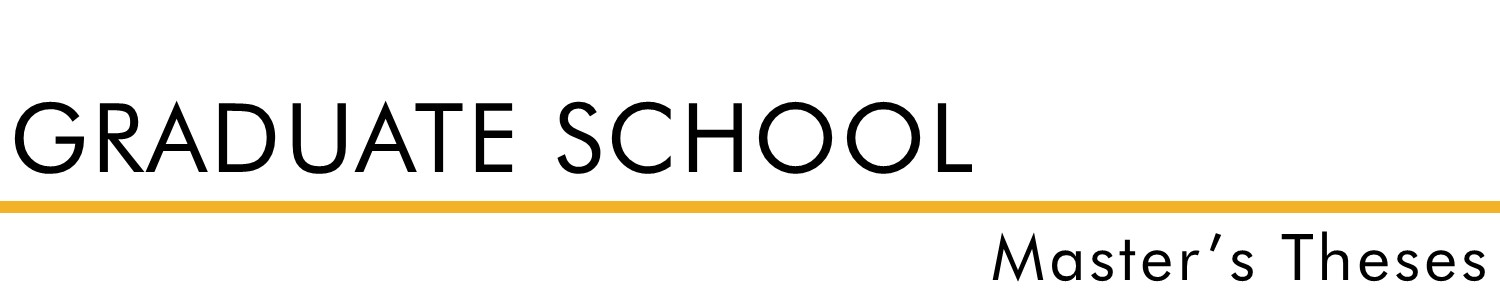#### Document Type

Thesis - campus only access

Spring 2007

#### Degree Name

Master of Science in Nursing (MSN)

Nursing

#### Advisor

Dr. Karyolyn Kells

#### Abstract

The nursing profession is experiencing a nursing shortage that is expected to only worsen. The current nursing work force is aging (Buerhaus, Staiger, & Auerbach, 2000), and fewer students overall are choosing nursing as a career (Mangan, 1999), and to compound the problem, the number of students unsuccessful on the National Council License Exam (NCLEX) for RNs is increasing. The purpose of this study was to determine relationships of variables with student results on the NCLEX-RN. The variables selected were (a) Nurse Entrance Test (Nurse Entrance Test [NET, 1990]) scores, (b) Admission Grade Point Average (GPA), (c) Nutrition first-time score, (d) growth and development (G & D) first-time scores, (e) Exiting GPA, (f) Assessment Technologies Institute exit examination (Assessment Technologies Institute [ATI, 1993]), (g) the Health Education Systems, Incorporated (Heath Education Systems, Inc. [HESI (E2)], 2001) exit examination, (h) the age of the student at program completion. A convenience sample consisted of AND graduate (N=158) first-time writers of the NCLEX-RN at a mid-western rural community college for the years from 2000-2006. Correlations were calculated using Spearman’s Rho. Research question number one was, “Is there a statistically significant relationship between the AND student’s NET score and he NCLEX-RN score?” The student NET scores were obtained from the AND program records. This question included the relationship of the Nurse Entrance Test (NET) (Skewness= -1.94, kurtosis= 5.80) and the NCLEX-RN score, pass or fail. The sample (n=140) graduates and NET scores of (M=61.82, SD = 16.08) were correlated with NCLEX-RN, rs=.286, p=.000. Research question number two was, “Is there a statistically significant relationship between the AND student’s admission GPA and the NCLEX-RN score?” This investigation included the relationship of the variables admission GPA (skewness= -1.70, kurtosis=6.70) and the NCLEX-RN score. There were (n=158) in the student sample and the admission GPA was found at (M=3.26, SD.55), rs=.258, p=.001. The third research question was, “Is there a statistically significant relationship between he AND student’s first grade in Nutrition and the NCLEX-RN?” This question included the relationship of the first-time Nutrition grade (skewness= -.75, kurtosis = -.026) variable and the NCLEX-RN score. There were (N=158) graduates of which the nutrition grades were found to have (M=3.27, SD=.76) and a Spearman’s Rho, rs=.148, p=.032. The fourth research question was, “Is there a statistically significant relationship between the AND student’s first-grade in G & D and the NCLEX-RN?” The G & D grades (M=3.46, SD=.76) were correlated with NCLEX-RN results, rs-.103, p=.099. The fifth research question was, “Is there a statistically significant relationship between the AND student’s exiting GPA and the NCLEX-RN?” This research question included the relationship between the existing GPA (skewness=11.99, kurtosis=148.42) and the NCLEX-RN score. There were (N=158) graduate records with (M=3.46, SD-2.433) correlated with NCLEX-RN results at, rs=.300, p=.000. The sixth research question was, “Is there a statistically significant relationship between the Assessment Technologies Institute (ATI) exit exam (skewness=-3.91, kurtosis=19.72) and the NCLEX-RN score?” Although the graduates of 2000 and 2001 (n=40) took the ATI, all data were not available for all students (n-35). The ATI scores (M=58.50, SC=11.58) were correlated with NCLEX-RN results using a Spearman’s RHO, rs=.536, p=.000. The seventh research question was, “Is there a statistically significant relationship between the Health Education System Inc. (HESI) exit exam (skewness=.322, kurtosis=-1.82) and the NCLEX-RN score?” There where (N=1.6) graduates from 2002 until the present with available HESI scores. The HESI scores (M=83.57, SC=11.49) were correlated with NCLEX-RN results, pass or fail, using a Spearman’s Rho, rs=.320, p=.000. The last research question of the investigation asked, “Is there a statistically significant relationship between the student’s age at the completion of the program and the NCLEX-RN score?” There (N=158) in the sample including the student’s age. The age (M=30.64, SD=8.50) were correlated with NCLEX-RN finding rs=.089, p=.132. The distribution was skewness=skewness=.876, kurtosis= .168. This investigation found there were academic variables that had statistically significant relationships at the investigational site. Three of these variables are standardized test that occur prior to the nursing program and after program completion.

#### Comments

Notice: This material may be protected by copyright law (Title 17 U.S. Code).

#### Rights

Copyright 2007 Jana K. Daigle

COinS